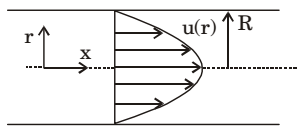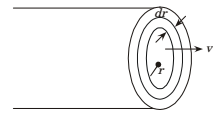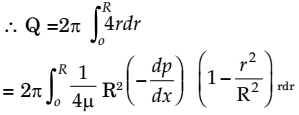## Fluid Mechanics and Hydraulic Machinery Miscellaneous

#### Fluid Mechanics and Hydraulic Machinery

1. Consider fully developed flow in a circular pipe with negligible entrance length effects. Assuming the mass flow rate, density and friction factor to be constant, if the length of the pipe is doubled and the diameter is halved, the head loss due to friction will increase by a factor of

1.  Head loss = fLv2 = fl(Q/A)2 2gd 2gd

 ⇒  Head loss ∝ L d5

 h1* ∝ L d5

 h2* ∝ 2.L = L × 64 (d/2)5 d5

 h1 = 1/64 ⇒ h2 = 64 h2 h1

##### Correct Option: D

 Head loss = fLv2 = fl(Q/A)2 2gd 2gd

 ⇒  Head loss ∝ L d5

 h1* ∝ L d5

 h2* ∝ 2.L = L × 64 (d/2)5 d5

 h1 = 1/64 ⇒ h2 = 64 h2 h1

1. Water is flowing through a horizontal pipe of constant diameter and the flow is laminar. If the diameter of the pipe is increased by 50% keeping the volume flow rate constant, then the pressure drop in the pipe due to friction will decrease by

1.  ∆ P = 32μUL ∆²

 Q = AV = π d²V 4

 V = 4Q πd²

 ∆P = 128μQL πD4

Above equation shows that
 ∆P ∝ 1 D4

 ∆P1 = C = C where D1 = D D41 D4

 & ∆P2 = C = C where D2 = 1.5D D42 (d42)

% change in pressure drop
 % = ∆P1 - ∆P2 × 100 ∆P1

= 80.24% ≃ 80%

##### Correct Option: D

 ∆ P = 32μUL ∆²

 Q = AV = π d²V 4

 V = 4Q πd²

 ∆P = 128μQL πD4

Above equation shows that
 ∆P ∝ 1 D4

 ∆P1 = C = C where D1 = D D41 D4

 & ∆P2 = C = C where D2 = 1.5D D42 (d42)

% change in pressure drop
 % = ∆P1 - ∆P2 × 100 ∆P1

= 80.24% ≃ 80%

1. The maximum velocity of a one-dimensional incompressible fully developed viscous flow, between two fixed parallel plates, is 6 ms-1. The mean velocity (in ms-1) of the flow is

1. For fully developed incompressible viscous flow,

 Vmean = 2 Vmax 3

 = 2 × 6 = 4 m/s 3

##### Correct Option: C

For fully developed incompressible viscous flow,

 Vmean = 2 Vmax 3

 = 2 × 6 = 4 m/s 3

1. The pressure drop for laminar flow of a liquid in a smooth pipe at normal temperature and pressure is

1.  ∆ P = 32μUL D²

Above equation shows that ∆P is independent of velocity.

##### Correct Option: C

 ∆ P = 32μUL D²

Above equation shows that ∆P is independent of velocity.

1. The velocity profile of a fully developed laminar flow in a straight circular pipe, as shown in the figure, is given by the expression
 u(r) = − R²∂p1 − r²4μ ∂x R²

where ∂p/∂x is a constant
The average velocity of fluid in the pipe is1.u = − R²− dp1 − r²4μ dx R

dQ =u (2πrdr)Solving, we get
 Q = − πR²− dp8μ dx

Now Q = Area ´ average velocity
 ∴  uav = Q = Q Area πR2

 or   uav = R²dp8μ dx

##### Correct Option: Au = − R²− dp1 − r²4μ dx R

dQ =u (2πrdr)Solving, we get
 Q = − πR²− dp8μ dx

Now Q = Area ´ average velocity
 ∴  uav = Q = Q Area πR2

 or   uav = R²dp8μ dx